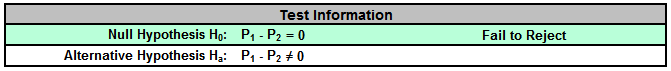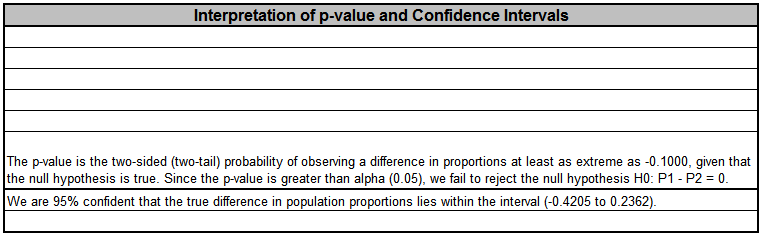Include Top

Basic Statistical Templates – 2 Proportions Test and Confidence Interval Example

Click SigmaXL > Templates & Calculators > Basic Statistical Templates > 2 Proportions Test and Confidence Interval to access the template.Notes
1. Enter summarized Sample Data, Null Hypothesis and Confidence Level in cells with yellow highlight. Do not modify any other part of this worksheet.
2. Select Alternative Hypothesis and Methods using drop-down.
3. Hypothesis Test Method:
• Fisher's Exact is recommended, but is only valid for H0: P1 - P2 = 0.
• Use Normal Approximation only when minimum expected cell value, shown above, is >= 5. Unpooled is used if H0: P1 - P2 <> 0. Use pooled if H0: P1 - P2 = 0. (See Peltier).
4. Confidence Interval Method:
• Confidence intervals for difference in binomial proportions have an "oscillation" phenomenon where the coverage probability varies with n and p.
• Newcombe-Wilson Score is recommended and has a mean coverage probability that is close to the specified confidence interval. (See Newcombe method 10).
• Newcombe-Wilson Score (CC = Continuity Corrected) is conservative and will typically meet the specified confidence level as a minimum coverage probability, but results in wider intervals. (See Newcombe method 11).
• Jeffreys-Perks is similar to Newcombe-Wilson, and included as an option because it is the preferred interval for some practitioners. (See Radhakrishna; Newcombe method 4).
• Normal Approximation is not recommended, but included here to validate hand calculations. Use only when minimum expected cell value, shown above, is >= 5.
• Exact methods that are strictly conservative (like Clopper-Pearson for the one proportion case) are not included in this template because they are computationally intensive and slow.
5. Due to the complexity of calculations, this template uses vba macros rather than Excel formulas. SigmaXL must be initialized and appear on the menu in order for this template to function.

## References:

Beal, S. L. (1987), Asymptotic confidence intervals for the difference between two binomial parameters for use with small samples. Biometrics, 43, 941-950.

Newcombe, R. G. (1998b), Interval estimation for the difference between independent proportions: Comparison of eleven methods. Statistics in Medicine, 17:873–890.

Peltier, C., Why Do We Pool for the Two-Proportion z-Test?, https://apcentral.collegeboard.com/apc/members/courses/teachers_corner/49013.html.

Radhakrishna S., Murthy B.N., Nair N.G., Jayabal P., Jayasri R. (1992), Confidence intervals in medical research. Indian J Med Res., Jun;96:199-205.

# Web Demos

Our CTO and Co-Founder, John Noguera, regularly hosts free Web Demos featuring SigmaXL and DiscoverSim